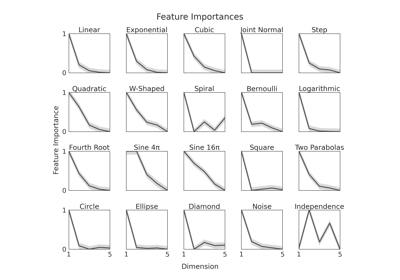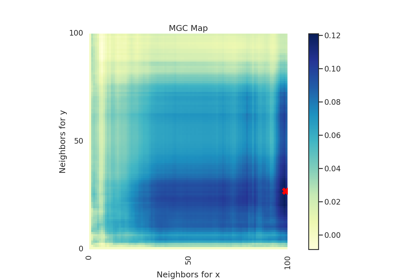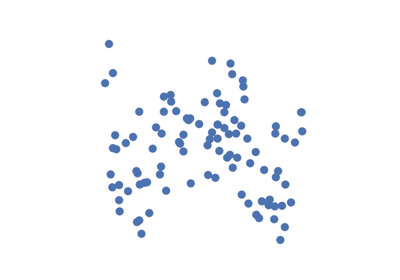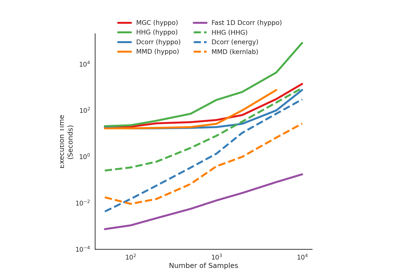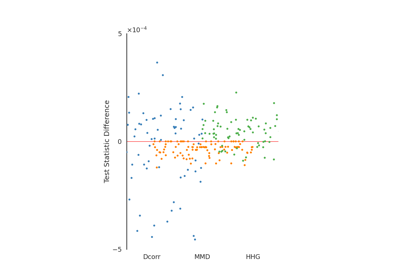# IndependenceTest¶

class hyppo.independence.base.IndependenceTest(compute_distance=None, **kwargs)

A base class for an independence test.

Parameters
• compute_distance (str, callable, or None, default: "euclidean" or "gaussian") -- A function that computes the distance among the samples within each data matrix. Valid strings for compute_distance are, as defined in sklearn.metrics.pairwise_distances,

• From scikit-learn: ["euclidean", "cityblock", "cosine", "l1", "l2", "manhattan"] See the documentation for scipy.spatial.distance for details on these metrics.

• From scipy.spatial.distance: ["braycurtis", "canberra", "chebyshev", "correlation", "dice", "hamming", "jaccard", "kulsinski", "mahalanobis", "minkowski", "rogerstanimoto", "russellrao", "seuclidean", "sokalmichener", "sokalsneath", "sqeuclidean", "yule"] See the documentation for scipy.spatial.distance for details on these metrics.

Alternatively, this function computes the kernel similarity among the samples within each data matrix. Valid strings for compute_kernel are, as defined in sklearn.metrics.pairwise.pairwise_kernels,

["additive_chi2", "chi2", "linear", "poly", "polynomial", "rbf", "laplacian", "sigmoid", "cosine"]

Note "rbf" and "gaussian" are the same metric.

• **kwargs -- Arbitrary keyword arguments for compute_distkern.

Methods Summary

 Calulates the independence test statistic. IndependenceTest.test(x, y[, reps, workers, ...]) Calulates the independence test statistic and p-value.

abstract IndependenceTest.statistic(x, y)

Calulates the independence test statistic.

Parameters

x,y (ndarray) -- Input data matrices. x and y must have the same number of samples. That is, the shapes must be (n, p) and (n, q) where n is the number of samples and p and q are the number of dimensions. Alternatively, x and y can be distance matrices, where the shapes must both be (n, n).

abstract IndependenceTest.test(x, y, reps=1000, workers=1, is_distsim=True, perm_blocks=None)

Calulates the independence test statistic and p-value.

Parameters
• x,y (ndarray) -- Input data matrices. x and y must have the same number of samples. That is, the shapes must be (n, p) and (n, q) where n is the number of samples and p and q are the number of dimensions. Alternatively, x and y can be distance matrices, where the shapes must both be (n, n).

• reps (int, default: 1000) -- The number of replications used to estimate the null distribution when using the permutation test used to calculate the p-value.

• workers (int, default: 1) -- The number of cores to parallelize the p-value computation over. Supply -1 to use all cores available to the Process.

• auto (bool, default: True) -- Automatically uses fast approximation when n and size of array is greater than 20. If True, and sample size is greater than 20, then hyppo.tools.chi2_approx will be run. Parameters reps and workers are irrelevant in this case. Otherwise, hyppo.tools.perm_test will be run.

• is_distsim (bool, default: True) -- Whether or not x and y are input matrices.

• perm_blocks (None or ndarray, default: None) -- Defines blocks of exchangeable samples during the permutation test. If None, all samples can be permuted with one another. Requires n rows. At each column, samples with matching column value are recursively partitioned into blocks of samples. Within each final block, samples are exchangeable. Blocks of samples from the same partition are also exchangeable between one another. If a column value is negative, that block is fixed and cannot be exchanged.

Returns

## Examples using hyppo.independence.base.IndependenceTest¶